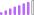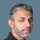cancel
Showing results for
Did you mean:Post Partisan

## Using icons in conditional formatting

Hi,

I'm trying to get icons displayed for Revision date column and using this measure which works fine:

CF1 =
VAR number_of_days =
SELECTEDVALUE ( 'MDR'[Revision date]) - TODAY ()
RETURN
SWITCH (
TRUE (),
number_of_days < 0, "Red",
number_of_days < 180, "Orange",
"Green"
)

But that only allows me to apply formatting by Field value which doesn't have the icon feature.
So I found this extra measure based on the previous one:

CF2 =
SWITCH(MAX([CF1]),
"Green",2,
"Yellow",1,
"Red",0
)

But it turns out CF1 has to be a column not a measure for the whole thing to work.
How do I convert CF1 from being a measure to a column?
Should I try a different approach?!

1 ACCEPTED SOLUTIONSuper User

Hey @Alex_0201 ,

I guess the revision date in the same table as the calculated column. Then try the following formula:

``````CF1 =
VAR number_of_days = 'MDR'[Revision date] - TODAY()
RETURN
SWITCH(
TRUE(),
number_of_days < 0, "Red",
number_of_days < 180, "Orange",
"Green"
)``````

Be aware that a calculated column is always static, so the value will be evaluated when the data model is loaded. After that the values stay as they are. They won't change when you change a slicer or something like that.

If you need any help please let me know.
If I answered your question I would be happy if you could mark my post as a solution ✔️ and give it a thumbs up 👍

Best regards
Denis

2 REPLIES 2Super User

@Alex_0201

You can do it with one measure, modify your measure as follows:

``````CF1 =
VAR number_of_days =
SELECTEDVALUE ( Table5[Date]) - TODAY ()
RETURN
SWITCH (
TRUE (),
number_of_days < 0, 1,
number_of_days < 180, 2,
3
)``````

Select the Date and Icon for CF:Configure CF as follows:Did I answer your question? Mark my post as a solution! and hit thumbs upSuper User

Hey @Alex_0201 ,

I guess the revision date in the same table as the calculated column. Then try the following formula:

``````CF1 =
VAR number_of_days = 'MDR'[Revision date] - TODAY()
RETURN
SWITCH(
TRUE(),
number_of_days < 0, "Red",
number_of_days < 180, "Orange",
"Green"
)``````

Be aware that a calculated column is always static, so the value will be evaluated when the data model is loaded. After that the values stay as they are. They won't change when you change a slicer or something like that.

If you need any help please let me know.
If I answered your question I would be happy if you could mark my post as a solution ✔️ and give it a thumbs up 👍

Best regards
Denis